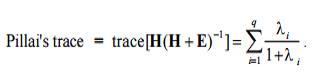# Pillai’s Trace

Multivariate Analysis > Pillai’s Trace

## What is Pillai’s Trace?

Pillai’s trace is used as a test statistic in MANOVA and MANCOVA. This is a positive valued statistic ranging from 0 to 1. Increasing values means that effects are contributing more to the model; you should reject the null hypothesis for large values.

Pillai’s is one of several test statistics used in MANOVA; Other commonly used tests include: Hotelling’s T2, Roy’s largest rootRoy’s Largest Root (Criterion): Definition and Wilks’ Lambda).

## When to use this test

This test is considered to be the most powerful and robust statistic for general use, especially for departures from assumptions. For example, if the MANOVA assumption of homogeneity of variance-covariance is violated, Pillai’s is your best option. It is also a good choice when you have uneven cell sizes or small sample sizes (i.e. when n is small).

However, when the hypothesis degrees of freedom is greater than one, Pillai’s tends to be less powerful than the other three. If you have a large deviation from the null hypothesis or the eigenvalues have large differences, Roy’s Maximum Root is a far better option (Seber 1984).

## Formula

The formula for Pillai’s trace is:The general steps for calculating the test statistic are:

1. Divide each eigenvalue by 1 + the characteristic root.
2. Sum these ratios.

It’s extremely rare that you would want to actually perform these calculations by hand; Most statistical packages will perform the calculations for you as part of MANOVA or MANCOVA. The output will return a Pillai’s trace value, associated F-statistic and a p-value. In general, small p-values (below .05) mean that Pillai’s returned a significant result (that there is a difference between the levels of independent variable you are looking at).

References:
Pillai K.C.S (1955). Some New test criteria in multivariate analysis. Ann Math Stat: 26(1):117–21.
Seber, G.A.F. (1984). Multivariate Observations. New York: John Wiley and Sons

CITE THIS AS:
Stephanie Glen. "Pillai’s Trace" From StatisticsHowTo.com: Elementary Statistics for the rest of us! https://www.statisticshowto.com/pillais-trace/
---------------------------------------------------------------------------Need help with a homework or test question? With Chegg Study, you can get step-by-step solutions to your questions from an expert in the field. Your first 30 minutes with a Chegg tutor is free!